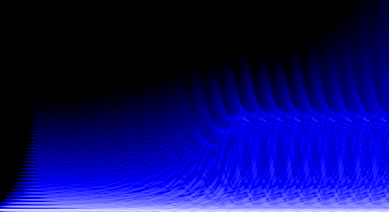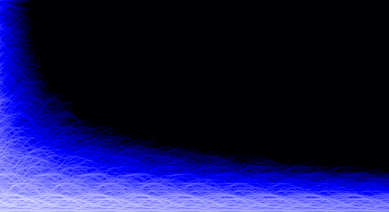# with audio-rate coefficient modulation

Jari Kleimola, Jussi Pekonen, Henri Penttinen, Vesa Välimäki

Dept. of Signal Processing and Acoustics

Helsinki University of Technology, TKK

Jonathan S. Abel

CCRMA, Dept. of Music

Stanford University

The example sounds from the paper can be found below. There are also some additional sounds which did not make it into the paper, but might be of interest otherwise. All sounds are 44.1 kHz 16-bit mono WAVs, normalized to -3 dB. The spectrograms at the top of this page were taken from the first two wavs, the rest of the sounds are in the order of appearance.

The software implementation with source code can be downloaded here. The zip file contains two Pure Data (PD) externals (ap~ is the chain, ksap~ is the string model, both compiled against PD v0.40.3-extended), C source, and a couple of example patches from the paper.

### Spectrogram sounds

• left figure: cascade - Two chains in a cascade, with dedicated modulators
• right figure: string - Simple physical string model, augmented with an allpass chain inside the model

### Synth sounds

• fig. 9: pseudo analog classics fx = 100 Hz, fx = 200 Hz - a) sawtooth c) square c) triangle
• fig. 10: cascade 1 - N1=73, N2=100, fx=200, fm1=299, fm2=33, M1=0->0.5, M2=0->0.99
• synth A: cascade 2 - N1=73, N2=1, fx=200, fm1=99, fm2=50, M1=0->0.99, M2=0.99
• synth B: frequency sweep - sweeping the modulator frequency
• synth C: 2-operator FM source - complex carrier, fm and M changed manually

### Augmented physical models and effects

all sounds zipped - 12.5 MB